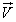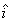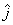This web app uses the HTML5 canvas element which is not supported by your browser. Please update your browser or try a different browser.

© 2014 Chris O'Donoghue. CC BY-NC 4.0 - profferings.ca

## Vector NotationDrag the purple vector by its tip.

### Magnitude-direction Notation

o.### Component (Unit Vector) Notation

The blue and red vectors are the horizontal and vertical components respectively of the purple vector.=+The Rectangular Coordinate System (RCS) is a particularly useful way of describing the direction of a vector.

In this system, zero degrees points to the right, i.e. along the positive horizontal axis, and the angle increases in a counter clockwise direction. So...

• straight up is 90o
• to the left is is 180o
• straight down is 270o ...and so on.

Angles can also be given as negative angles, ie. measured in the clockwise direction. So, for example, 270o, i.e. 270o anti-clockwise from zero, is the same direction as -90o, i.e. 90o clockwise from zero. It is common to give the directions of vectors in the 4th quadrant as negative directions.

Drag the vector at left to illustrate this.

Unit vector notation makes it easy to work with components of vectors and to write down what you are doing.

A unit vector has a length of 1. The symbolrepresents a horizontal unit vector, i.e. a vector 1 unit long pointing along the positive x-axis. A vector 3 units long pointing to the right could be written as 3. Vectors pointing to the left have negative magnitudes, so 6.2 units to the left can be writen as -6.2.

Similarlyrepresents a vertical unit vector. 5is 5 units long pointing up and -3is 3 units long pointing down.

A vector 5 units long in a direction of 127o can be thought of as a combination of 3 units to the left plus 4 units up. We can write this as -3+ 4.

The draggable purple vector at left is+